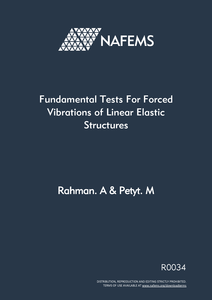This Website is not fully compatible with Internet Explorer.
For a more complete and secure browsing experience please consider using Microsoft Edge, Firefox, or Chrome

## Fundamental Tests For Forced Vibrations of Linear Elastic StructuresThis report presents a set of benchmark tests for the dynamic response of Linear elastic structures.

The benchmark tests include:

• Deep simply supported beam
• Thin simply-supported rectangular beam
• Thick simply-supported rectangular beam

Responses to harmonic, periodic, transient and random forces are presented. In each case response quantities such as displacement, velocity, acceleration and stresses are calculated at selected locations on the structure. Both tabular and graphical presentation of the results in frequency or time domains are given.

The report describes in depth the analytical/reference theory for the solution of the equations of motion for the free vibration and dynamic response of each structure. This has been incorporated in software programs to produce results for selected test examples.

Results from typical finite element models for the same test examples are also presented for each case.

## Contents

Introduction

• Background
• Requirements
• Structural Configurations
• Response Quantities and Locations
• Report layout

Dynamic Response of Deep Simply-Supported Beam

• Reference Solution
• General Theory
• Harmonic Response
• Periodic Response
• Transient Response
• Random Response
• Test Example
• Free Vibration Analysis
• Finite Element Models and Solution
• Test Examples
• Free Vibration Analysis
• Accuracy of Reference and Finite Element Solutions
• Reference Solution
• Finite Element Solution

Dynamic Response of Thin Simply-Supported Rectangular Plate

• Reference Solution
• General theory
• Test Examples
• Free Vibration Analysis
• Finite Element Models and Solutions
• Test Examples
• Free Vibration Analysis

Dynamic Response of Thick Simply-Supported Rectangular Plate

• Reference Solution
• General Theory
• Test Example
• Free Vibration Analysis
• Finite Element Models and Solution
• Test Examples
• Free Vibration Analysis

REFERENCES

TABLES

FIGURES

APPENDIX 1 Free Vibration of a Deep Beam

APPENDIX 2 Transient Response of a Single Degree of Freedom System to Harmonic Excitation

APPENDIX 3 Free Vibration of a Thin Plate

APPENDIX 4 Free Vibration of a Thick Plate

### Document Details

Reference R0034 Rahman. A Petyt. M English Analyst Developer Publication 1st January 1994 Global